# Square Wave VI

## LabVIEW 2018 Help

Edition Date: March 2018
Part Number: 371361R-01
View Product InfoLabVIEW 2015 HelpLabVIEW 2016 HelpLabVIEW 2017 HelpLabVIEW 2018 HelpLabVIEW 2019 Help

Owning Palette: Signal Generation VIs

Requires: Full Development System

Generates an array containing a square wave.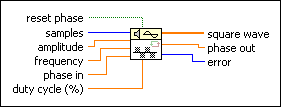reset phase determines the initial phase of square wave. The default is TRUE. If reset phase is TRUE, LabVIEW sets the initial phase to phase in. If reset phase is FALSE, LabVIEW uses the value of phase out from when the VI last executed as the initial phase of square wave.samples is the number of samples of the square wave. The default is 128.amplitude is the amplitude of square wave. The default is 1.0.frequency is the frequency of square wave in normalized units of cycles/sample. The default is 1 cycle/128 samples or 7.8125E–3 cycles/sample.phase in is the initial phase, in degrees, of square wave when reset phase is TRUE. The default is 0.duty cycle is the percentage of time a square wave remains high versus low over one period. The default is 50.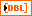square wave is the output square wave.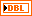phase out is the phase, in degrees, of the next sample of square wave.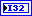error returns any error or warning from the VI. You can wire error to the Error Cluster From Error Code VI to convert the error code or warning into an error cluster.

## Square Wave Details

If the sequence Y represents square wave, the Square Wave VI generates the pattern according to the following equation.

yi = a*square(phase[i])

for i = 0, 1, …, n – 1 and where a is amplitude and n is the number of samples.

This VI calculates square(phase[i]) using the following equation: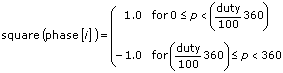,

where

p = phase[i] modulo 360,

duty is duty cycle,

phase[i] = initial_phase + frequency*360*i,

frequency is the frequency in normalized units of cycles/sample,

initial_phase is phase in if reset phase is TRUE,

initial_phase is the phase out from the previous execution of this instance of the VI if reset phase is FALSE.

The Square Wave VI is reentrant, so you can use it to simulate a continuous acquisition from a square wave function generator. If the input control reset phase is FALSE, subsequent calls to a specific instance of the Square Wave VI produce the output square wave array containing the next samples of a square wave.

Because the Square Wave VI is reentrant, if reset phase is FALSE, the VI uses the phase out value as its new phase in the next time the VI executes.

## Example

Refer to the Function Generator with FM VI in the labview\examples\Signal Processing\Signal Generation directory for an example of using the Square Wave VI.